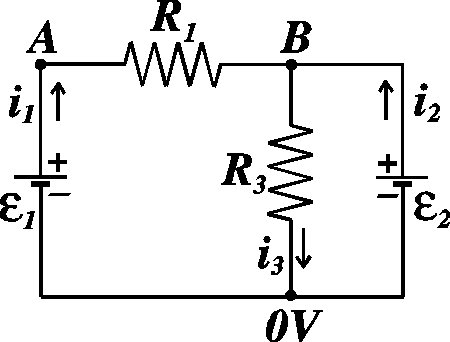# Find and calculate current in a circuit

choco_moo

## Homework Statement

Consider the following configuration.Given:
EMF1=6 V
EMF2 = 3 V
R1 = 600 Ohm
R3 = 100 Ohm

1. Calculate the current i1.
2. Calculate the current i3.

## Homework Equations

V=IR
Kirchoff's circuit laws:
1. Sum of current into any node is zero.
2. Sum of voltages around any loop is zero.

## The Attempt at a Solution

I know that the potential at point A is 6 V because of the battery. I can't figure out how to calculate i1. I think I somehow have to use Kirchoff's rule, but I just don't know how. I tried to set up a voltage equation: EMF1 -i1R1 - i3R3 = 0. I don't know if that's right but it still has two unknowns. For i3, it seems like I have to know what i1 is to calculate it. Any help is appreciated!

## Answers and Replies

Homework Helper
This voltage equation: EMF1 -i1R1 - i3R3 = 0. is correct.
Write down similar equation for EMF2. Put i3 = i1 + i2 and solve the equations to find i1 and i2.

choco_moo
How would I know which resistor i2 corresponds to?

Homework Helper
EMF2 - (i1 + i2)R3 = 0.

choco_moo
Ah, that makes sense. So I set those two voltage equations equal to each other and solved for i1. Then I plugged i1 into the EMF2 voltage equation and solved for i2. I finally added i1 and i2 together to get i3. Thanks so much!